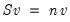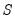Command Reference : Matrix Language Reference

 @eigenvectors
Eigenvectors of symmetric matrix.
Syntax: @eigenvectors(s)
s: sym
Return: matrix
Returns a square matrix whose columns are the eigenvectors of the sym s.
Each eigenvector v satisfies, whereis the symmetric matrix given by s, and where n is the eigenvalue associated with the eigenvector v. The eigenvalues are arranged in ascending order, and the columns of the eigenvector matrix correspond to the sorted eigenvalues.
Examples
sym s1 = @unvech(@mnrnd(15))
matrix m2 = @eigenvectors(s1)
Cross-references
See @eigenvalues.